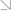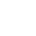全国热线：400-963-0708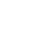网站地图 Sign in Sign up

# 本科学历 + CFA + python，对从事金融行业有什么帮助？01

01

02

03

02

# 金融行业中的具体应用？

### 1.金融和Python语法

（1）从标准正态分布中取得I个（伪）随机数z(i)，i∈{1，2，…，I}。

（2）为给定的z(i)和公式1-1计算所有到期指数水平ST(i)。

（3）计算到期时期权的所有内在价值hT(i)=max(ST(i)−K,0)。

（4）通过公式1-2中给出的蒙特卡罗估算函数估计期权现值。

``````In : import math
import numpy as np ❶

In : S0 = 100. ❷
K = 105. ❷
T = 1.0 ❷
r = 0.05 ❷
sigma = 0.2 ❷

In : I = 100000 ❷

In : np.random.seed(1000) ❸
In : z = np.random.standard_normal(I) ❹

In : ST = S0 * np.exp((r - sigma ** 2 / 2) * T + sigma * math.sqrt(T) * z) ❺

In : hT = np.maximum(ST - K, 0) ❻

In : C0 = math.exp(-r * T) * np.mean(hT) ❼

In : print('Value of the European call option: {:5.3f}.'.format(C0)) ❽
Value of the European call option: 8.019.``````

### 2.Python的效率和生产率

Python效率较为明显的领域之一是交互式的数据分析这些领域从IPython、Jupyter Notebook等有力工具和pandas之类的程序中库获益良多。

``````In : import numpy as np ❶
import pandas as pd ❶
from pylab import plt, mpl ❷

In : plt.style.use('seaborn') ❷
mpl.rcParams['font.family'] = 'serif' ❷
%matplotlib inline

index_col=0, parse_dates=True) ❸
data = pd.DataFrame(data['.SPX']) ❹
data.dropna(inplace=True) ❹
data.info() ❺
<class 'pandas.core.frame.DataFrame'>
DatetimeIndex: 2138 entries, 2010-01-04 to 2018-06-29
Data columns (total 1 columns):
.SPX 2138 non-null float64
dtypes: float64(1)
memory usage: 33.4 KB

In : data['rets'] = np.log(data / data.shift(1)) ❻
data['vola'] = data['rets'].rolling(252).std() * np.sqrt(252) ❼
In : data[['.SPX', 'vola']].plot(subplots=True, figsize=
(10, 6)); ❽``````

### 3.人工智能优先金融学

TensorFlow当然和股票自动算法交易一点关联都没有，但却可以用于预测金融市场的变动，最广泛使用的Python ML程序库是scikit-learn。

``````In : import numpy as np
import pandas as pd

index_col=0, parse_dates=True)
data = pd.DataFrame(data['AAPL.O']) ❶
data['Returns'] = np.log(data / data.shift()) ❷
data.dropna(inplace=True)

In : lags = 6

In : cols = []
for lag in range(1, lags + 1):
col = 'lag_{}'.format(lag)
data[col] = np.sign(data['Returns'].shift(lag)) ❸
cols.append(col)
data.dropna(inplace=True)``````

❶ 选择苹果公司（AAPL.0）历史日终数据。
❷ 计算整个历史数据内的对数收益率。
❸ 生成带有定向滞后对数收益率数据（+1或者−1）的DateFrame列。

03

对金融行业有什么帮助？

Python3.0全新升级

#Python金融时代已经到来#CFA学习交流群(扫码免费加群)

•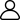1、新手入门
•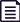2、学习资料
•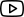3、免费课程
•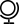4、考试动态
•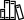5、备考干货
•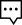6、答疑冲刺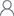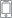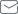2021年8月13日CFA一级考试 还有

81
18小时 45分钟 36

## 推荐课程